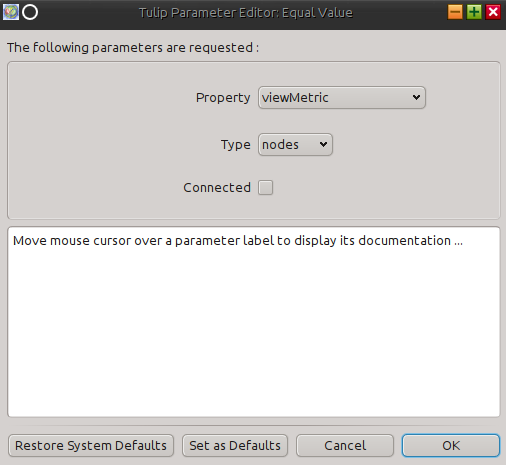Warning

If you are using the bindings through the classical Python interpreter, Tulip plugins must be loaded in order to be able to call algorithms (see Loading Tulip plugins)).

# Applying an algorithm on a graph¶

In Tulip, algorithms are implemented as plugins. There is two main type of algorithms :

• General : algorithms of this type can work on the input graph in a free manner and perform a variety of tasks (modifying the graph structure, creating graph properties, ...).
• Property : algorithms of this type can only compute data to attach on the graph elements and fill an output graph property (e.g. layout algorithm, metric algorithm, ...).

In the remaining of this section, you will learn how to call each type of algorithm from Python but also how to transmit parameters to the algorithms.

Important

As algorithms are plugins, they must be loaded before you can call them. If you use the bindings from the Tulip GUI, all installed plugins are already loaded. If you use the bindings from the Python shell, you need to manually load the plugins (see Using the bindings from the Python Interpreter).

## Calling a general algorithm on a graph¶

To call a general algorithm, you must use the tlp.Graph.applyAlgorithm() method. From the Tulip GUI, you can see all the general algorithms currently loaded by looking at the entries from the Algorithm -> General menu. Alternatively, from Python you can get a list of the general algorithms names by calling the tlp.getAlgorithmPluginsList() function.

As an example, we will call the “Equal value” algorithm from Python. This algorithm takes an input graph property and partitions the graph elements (nodes or edges) according to the values of the property. More precisely, all graph elements having the same property value are grouped in a class. Then for each class, a sub-graph is created.

This algorithm takes 3 parameters as illustrated in the screenshot below:Below is a description of each parameter :

• Property : the input graph property of type tlp.PropertyInterface from which to compute the partition
• Type : a tlp.StringCollection parameter to choose the type of the elements to partition (two possible values : “nodes” or “edges”)
• Connected : a boolean parameter indicating whether the output sub-graphs have to be connected

The sample code below illustrates how to call this algorithm from Python. We assume that a Tulip graph is bound to a “graph” variable and this graph contains a double property called “myMetric”:

```# get the input property from which to generate the partition
myMetric = graph.getDoubleProperty("myMetric")

# get a data set filled with default parameters for the algorithm
dataSet = tlp.getDefaultPluginParameters("Equal Value", graph)

# set the input property
dataSet["Property"] = myMetric

# if you want to partition edges instead of nodes
# dataSet["Type"].setCurrent(1) # use string collection index
# or alternatively
# dataSet["Type"].setCurrent("edges") # use string collection value

# if you want connected sub-graphs
# dataSet["Connected"] = True

# now we call the algorithm
graph.applyAlgorithm("Equal Value", dataSet)
```

## Calling a property algorithm on a graph¶

To call a property algorithm on a graph, you must use specific methods from the tlp.Graph class. There is one method per type of property to compute like for instance tlp.Graph.applyBooleanAlgorithm(), tlp.Graph.applyLayoutAlgorithm(), ...

As an illustration, we will call the “Hierarchical Graph” layout algorithm, that can be used to draw a tree.

This algorithm takes 4 parameters as illustrated in the screenshot below:Below is a description of each parameter :

• node size : an input size property of type tlp.SizeProperty that contains nodes sizes
• orientation : a tlp.StringCollection parameter to choose the layout orientation (two possible values : “horizontal” or “vertical”)
• layer spacing : a float parameter to set up the minimum distance between two layers in the drawing
• node spacing : a float parameter to set up the minimum distance between two nodes in the same layer

The sample code below illustrates how to call this layout algorithm from Python. We assume that a Tulip graph is bound to a “graph” variable:

```# get a data set filled with default parameters for the algorithm
dataSet = tlp.getDefaultPluginParameters("Hierarchical Graph", graph)

# set the orientation to vertical
dataSet["orientation"].setCurrent("vertical")

# set custom spacing
dataSet["layer spacing"] = 100.0
dataSet["node spacing"] = 50.0

# get a reference to the default layout property
viewLayout = graph.getLayoutProperty("viewLayout")

# call the layout algorithm and store the result in viewLayout
graph.applyLayoutAlgorithm("Hierarchical Graph", viewLayout, dataSet)
```